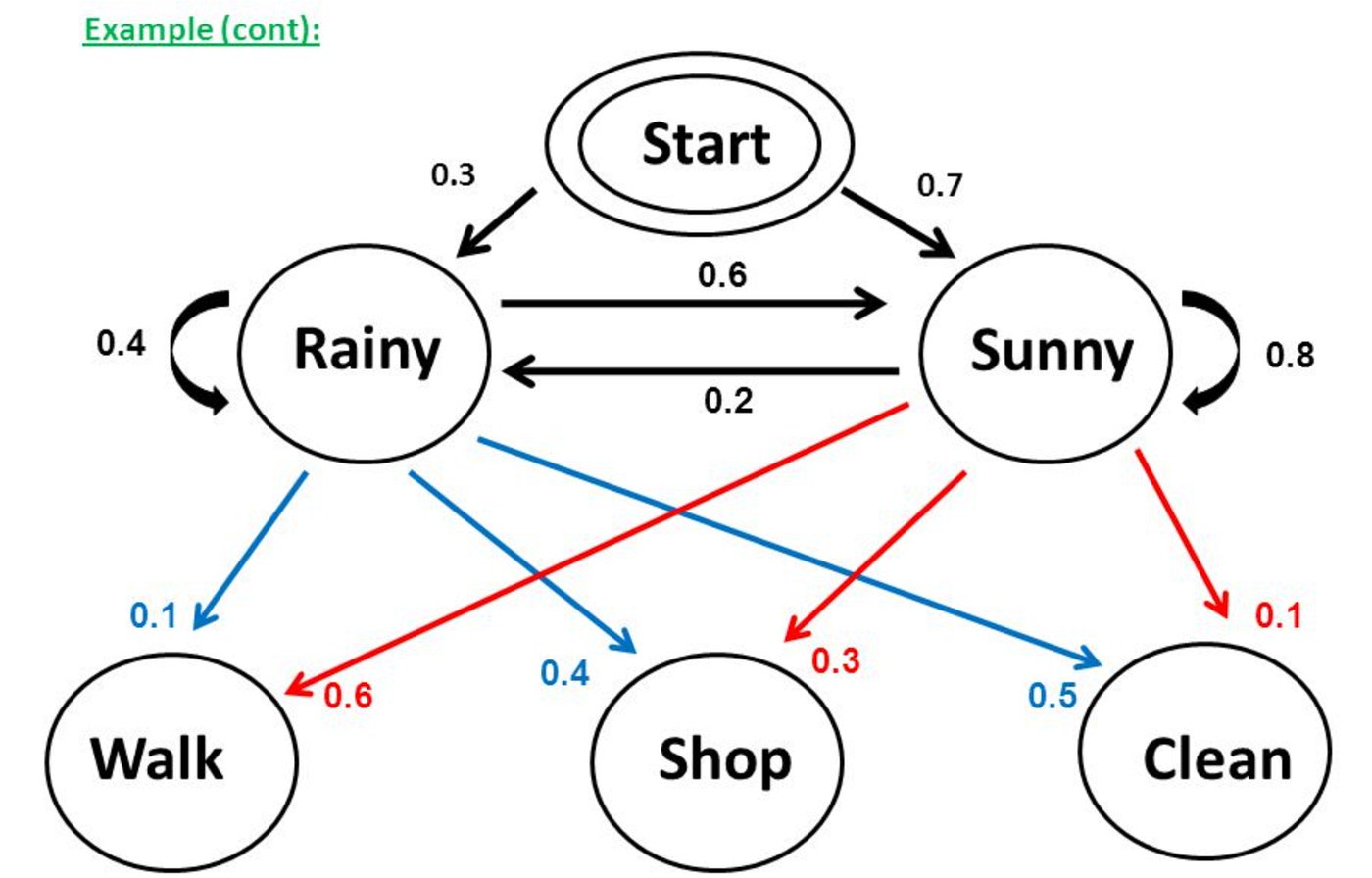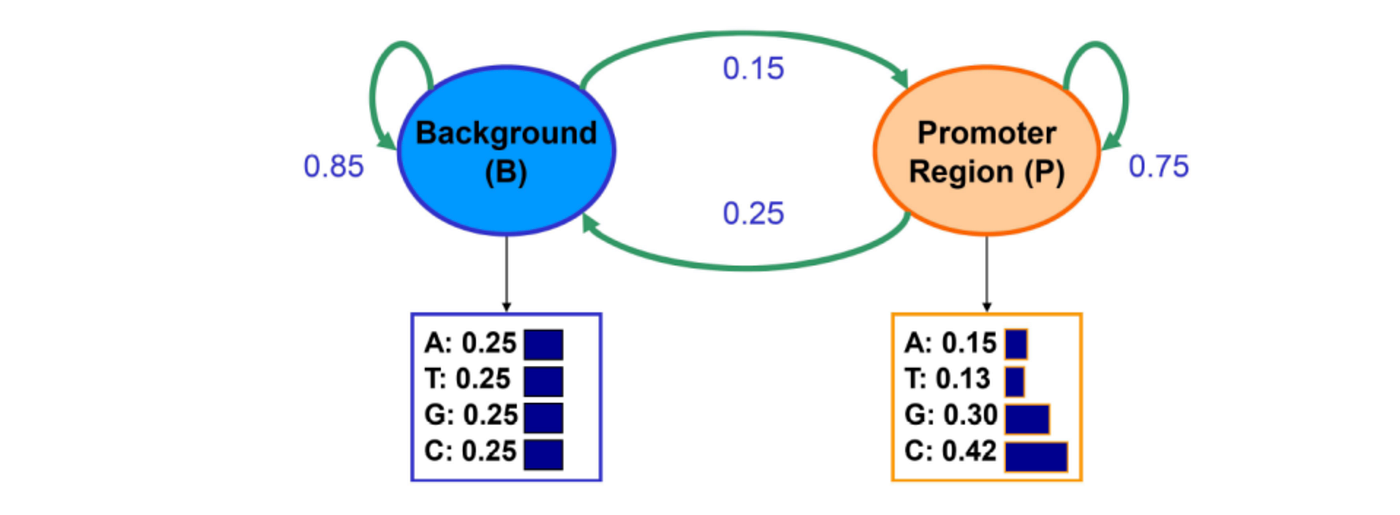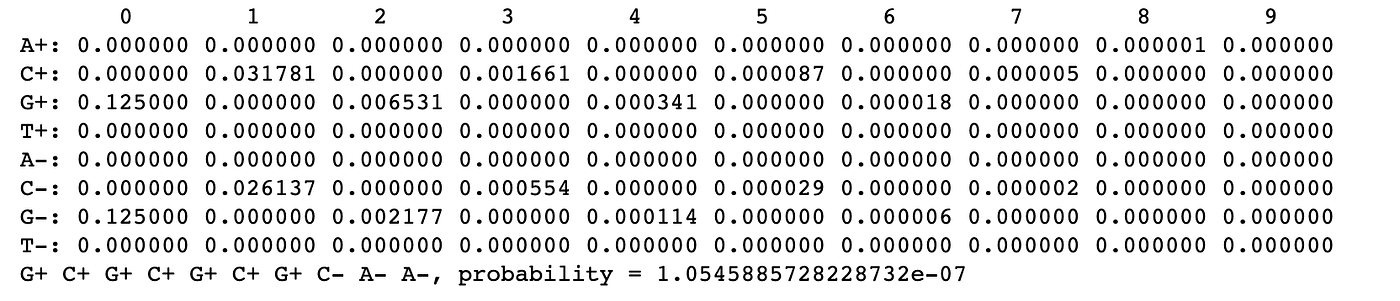# Identifying Promoter Regions Using the Viterbi Algorithm

• Promoter regions: regions upstream or next to where a gene will be transcribed that RNA polymerase binds to
• Coding regions: the portion of the DNA or RNA that codes for proteins
• Termination region: regions downstream that signal for RNA polymerase to break off

# Hidden Markov ModelsExample of a Hidden Markov Model.
• Observations/emissions: These are the observations that the user is giving to the model. For this particular model, these would be “Walk”, “Shop”, and “Clean”.
• States: These are the “hidden” values that we are trying to infer from the observations using probability analysis. For this particular model, these would be “Rainy” and “Sunny”.
• Initial probabilities: These are the probabilities that the states will take place in the first step. One such example is that there is a 30% chance of starting at the Rainy state. In other words, P(Rainy) = 0.3.
• Transition probabilities: These are the probabilities within various states. One such example is the one aforementioned about Rainy to Sunny. Assuming t_k is the current state, we can say P(t_k+1 = “Sunny” | t_k = “Rainy”) = 0.6.
• Emission probabilities: These are the probabilities that a state will cause this particular emission. For instance, P(Shop | Rainy) = 0.4.

## Converting to Code

`from Bio.HMM.MarkovModel import HiddenMarkovModeltransition_alphabet = ['Rainy', 'Sunny']emission_alphabet = ['W', 'S', 'C'] # W - walk, S - shop, C - cleaninitial_prob = {'Rainy': 0.3, 'Sunny': 0.7}transition_prob = {('Rainy', 'Rainy'): 0.4,                 ('Rainy', 'Sunny'): 0.6,                 ('Sunny', 'Rainy'): 0.2,                 ('Sunny', 'Sunny'): 0.8}emission_prob = {('Rainy', 'W'): 0.1,                 ('Rainy', 'S'): 0.4,                 ('Rainy', 'C'): 0.5,                 ('Sunny', 'W'): 0.6,                 ('Sunny', 'S'): 0.3,                 ('Sunny', 'C'): 0.1}model = HiddenMarkovModel(transition_alphabet, emission_alphabet, initial_prob, transition_prob, emission_prob, 1, 1)`

## Viterbi Algorithm

`model.viterbi(Seq('WSC'), transition_alphabet)`
`(Seq('SunnySunnyRainy'), -4.5972020163389145)`

# Identifying Promoter RegionsExample of Hidden Markov Model in biology.
`from Bio.HMM.MarkovModel import HiddenMarkovModeltransition_alphabet = ['B', 'P'] # B - background, P - promoteremission_alphabet = ['A', 'T', 'C', 'G']initial_prob = {'B': 0.5, 'P': 0.5}transition_prob = {('B', 'B'): 0.85,                  ('B', 'P'): 0.15,                  ('P', 'P'): 0.75,                  ('P', 'B'): 0.25}emission_prob = {('B', 'A'): 0.25,                ('B', 'T'): 0.25,                ('B', 'C'): 0.25,                ('B', 'G'): 0.25,                ('P', 'A'): 0.15,                ('P', 'T'): 0.13,                ('P', 'C'): 0.42,                ('P', 'G'): 0.3}model = HiddenMarkovModel(transition_alphabet, emission_alphabet, initial_prob, transition_prob, emission_prob, 1, 1)`
`model.viterbi(Seq('AGTACACTGGT'), transition_alphabet)model.viterbi(Seq('GCGCGCGCAA'), transition_alphabet)`
`(Seq('BBBBBBBBBBB'), -17.567574447856494)(Seq('PPPPPPPPBB'), -15.314217188702496)`

# HMMs with “Memory”

`array_promoter = [0.18, 0.274, 0.426, 0.12, 0.171, 0.368, 0.274, 0.188, 0.161, 0.339, 0.375, 0.125, 0.079, 0.355, 0.384, 0.182]vals_pp = [0.75*val for val in array_promoter]vals_pb = [0.25*val for val in array_promoter]array_background = [0.3, 0.205, 0.285, 0.21, 0.322, 0.298, 0.078, 0.302, 0.248, 0.246, 0.298, 0.208, 0.177, 0.239, 0.292, 0.292]vals_bp = [0.15*val for val in array_background]vals_bb = [0.85*val for val in array_background]`
`model.viterbi(Seq('AGTACACTGGT'), transition_alphabet)model.viterbi(Seq('GCGCGCGCAA'), transition_alphabet)`
`(Seq('A-G-T-A-C-A-C-T-G-G-T-'), -17.77362274426406)(Seq('G+C+G+C+G+C+G+C-A-A-'), -16.064944938458574)`

# Viterbi Algorithm

`def viterbi_algorithm(observations, states, start_p, trans_p, emit_p, table):    V = [{}]        for st in states:        V[st] = {"prob": start_p[st] * emit_p[st][observations], "prev": None}`
`for t in range(1, len(observations)):        V.append({})        for st in states:            max_tr_prob = V[t - 1][states]["prob"] * trans_p[states][st]            prev_st_selected = states             for prev_st in states[1:]:                tr_prob = V[t - 1][prev_st]["prob"] * trans_p[prev_st][st]                if tr_prob > max_tr_prob:                    max_tr_prob = tr_prob                    prev_st_selected = prev_st             max_prob = max_tr_prob * emit_p[st][observations[t]]            V[t][st] = {"prob": max_prob, "prev": prev_st_selected}`
`opt = []max_prob = 0.0best_st = None for st, data in V[-1].items():    if data["prob"] > max_prob:        max_prob = data["prob"]        best_st = st    opt.append(best_st)    previous = best_st`
`for t in range(len(V) - 2, -1, -1):        opt.insert(0, V[t + 1][previous]["prev"])        previous = V[t + 1][previous]["prev"]print (" ".join(opt) + ", probability = %s" % max_prob)`Final result of the self-coded Viterbi algorithm.
`HiddenMarkovModel ImplementationG+C+G+C+G+C+G+C-A-A-, probability = -16.064944938458574Own ImplementationG+C+G+C+G+C+G+C-A-A-, probability = 1.0545885728228732e-07`

# TL;DR

• Hidden Markov Models have both states and emissions, which are interconnected by conditional probability scores.
• Hidden Markov Models are a tool used to infer whether a region is a promoter or background region based on a sequence of nucleotides.
• The Viterbi algorithm is a dynamic programming algorithm that allows for this process to occur by looking at various transition and emission probabilities and keeping a pointer matrix to trace back through.

--

--